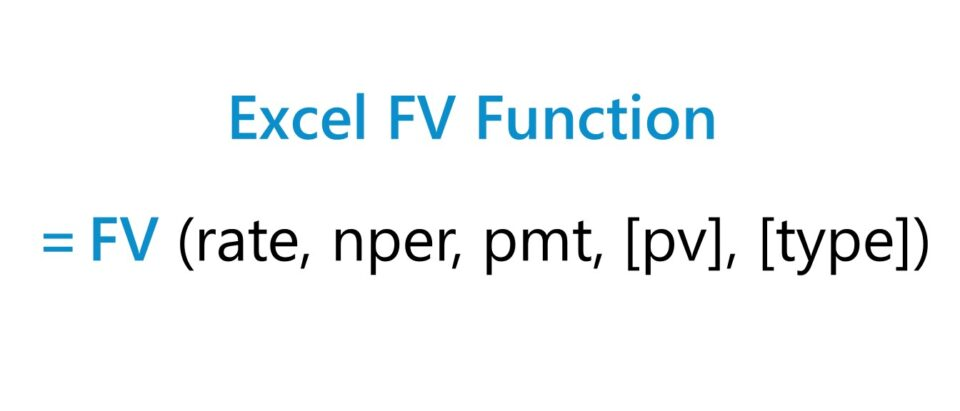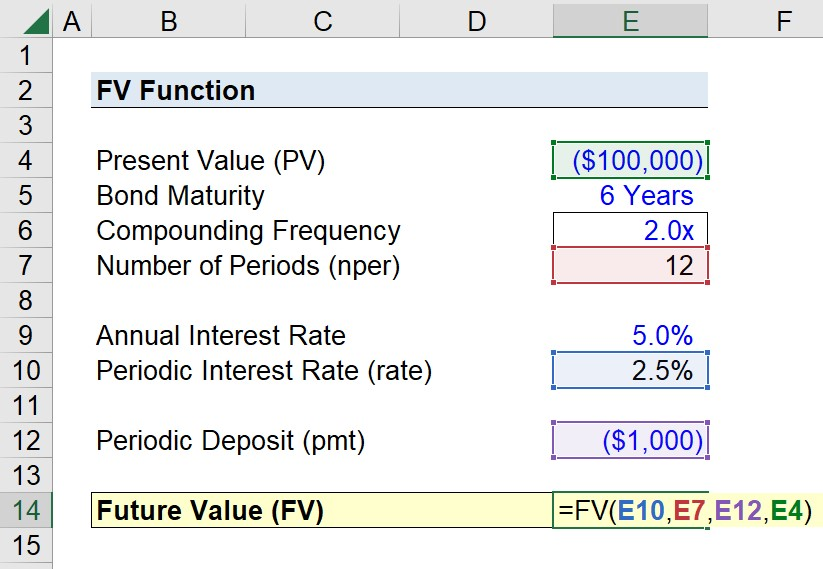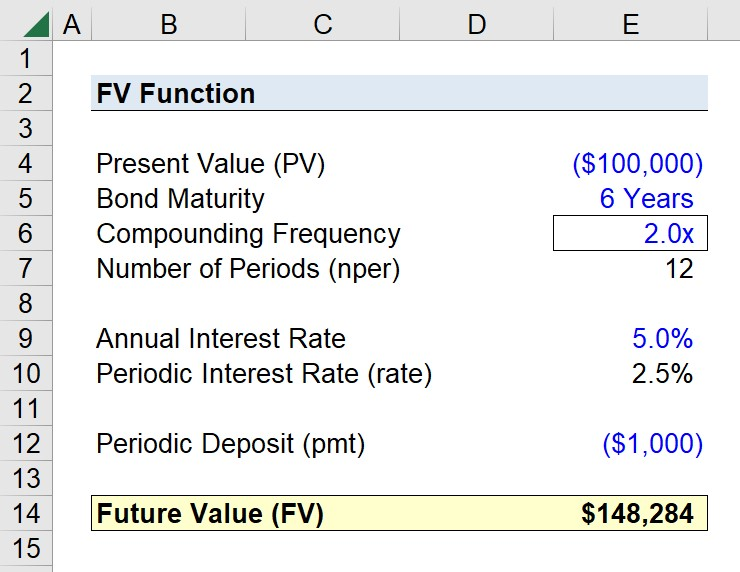Welcome to Wall Street Prep! Use code at checkout for 15% off.# FV Function

Guide to Understanding the Excel FV Function## How to Use FV Function in Excel?

The Excel FV function is a built-in feature used to determine the future value of a series of cash flows, i.e. how much a series of cash flows is expected to be worth on a future date.

The future value (FV) along with the present value (PV) are two fundamental concepts in corporate finance and valuation.

These concepts are tied to the principle of the “time value of money,” i.e. a dollar received today is worth more than a dollar received on a future date.

Hence, a future cash flow must be discounted back to the present date using an appropriate discount rate. The discount rate represents the expected return on an investment, with respect to the riskiness of the future cash flow(s).

However, please note that the Excel FV function is only appropriate if the series of future cash flows remain consistent over time – i.e. periodic or constant payments with a fixed interest rate – or consists of a lump sum payment.

## FV Function Formula Syntax

The formula to use the FV function in Excel is as follows.

=FV(rate,nper,pmt,[pv],[type])
• rate” → Interest Rate (%)
• nper” → Total Number of Payment Periods
• pmt” → Periodic Payment
• pv” → Present Value
• type” → Timing of Payment (0 = Payment Due at End of Period; 1 = Payment Due at Beginning of Period)

The “fv” and “type” arguments are enclosed in brackets because they are optional inputs that can be omitted, i.e. left blank.

1. Present Value → If the “pv” argument is omitted, the assumption is that the value of the investment on the initial date is zero. The “pmt” argument is required if the “pv” argument is omitted, but can otherwise be left blank (or there can also be values entered for both arguments).
2. Timing of Payment → If the “type” argument is omitted, Excel will automatically use its default setting, which assumes the payments come due at the end of each period.

## Periodicity Conversion Chart: “rate” and “nper” Adjustment

The “rate” and “nper” arguments – the interest rate and the total number of payments periods – must be consistent with regard to timing.

The following table outlines how to adjust annual rates to match various timeframes:

Compounding Frequency “rate” “nper”
Weekly
• ÷ 52
• × 52
Monthly
• ÷ 12
• × 12
Quarterly
• ÷ 4
• × 4
Semi-Annual
• ÷ 2
• × 2
Annual
• NA
• NA

## Excel FV Function Error Message (#VALUE!)

If an error message results from the FV function, the most common mistake is the sign convention.

In order for the Excel FV function to work as intended, the arguments that result in an “inflow” of cash should be entered as a positive number, whereas those that represent an “outflow” of cash must be entered as a negative number.

## FV Function Calculator — Excel Model Template

We’ll now move on to a modeling exercise, which you can access by filling out the form below.Submitting...

## Step 1. Future Value of Bond Assumptions (FV)

Suppose you’re tasked with calculating the future value (FV) of a semi-annual corporate bond.

On the date of issuance, the present value (PV) of the corporate bond was \$100,000 with a maturity of 6 years and an annual coupon rate of 5.0%.

The bond assumptions stated thus far are summarized here:

• Present Value (PV) = \$100,000
• Bond Maturity = 6 Years
• Compounding Frequency = 2.0x (Semi-Annual Bond)
• Annual Interest Rate = 5.0%

## Step 2. “nper” and “rate” Adjustment + “pmt” Assumption

In the next step, we’ll adjust the periodicity of each input to ensure that all of the inputs are consistent in terms of timing. The assumptions regarding the bond maturity and interest rate were denoted on an annual basis, so it is necessary to convert them into a semi-annual basis.

• Number of Periods (nper) = 6 Years × 2 = 12 Periods
• Periodic Interest Rate (rate) = 5.0% ÷ 2 = 2.5%

The remaining input is the periodic deposit payment, which we’ll assume to be \$1,000. Given that there are 12 periods, the total deposits across the bond’s maturity will amount to \$12,000.

As mentioned earlier, be sure to confirm that the “pv” and “pmt” arguments are entered as negative numbers because they represent an outflow of cash (or manually place the negative sign in the final equation).

## Step 3. FV Function in Excel Calculation Example

Now that we’ve converted the periodicity of the arguments, the final step is to use the Excel FV function to compute the future value (FV).

=FV(E10,E7,E12,E4)The “type” argument was intentionally left blank, since we’ll assume the payments come due at the end of each period.

In closing, we arrive at a future value (FV) of \$148,284 for the corporate bond given our set of assumptions.Turbo-charge your time in Excel Used at top investment banks, Wall Street Prep's Excel Crash Course will turn you into an advanced Power User and set you apart from your peers.